Related Articles

# Filtering rows based on column values in PySpark dataframe

• Last Updated : 29 Jun, 2021

In this article, we are going to filter the rows based on column values in PySpark dataframe.

## Python3

 `# importing module``import` `spark`` ` `# importing sparksession from pyspark.sql module``from` `pyspark.sql ``import` `SparkSession`` ` `# creating sparksession and giving an app name``spark ``=` `SparkSession.builder.appName(``'sparkdf'``).getOrCreate()`` ` `# list  of employee data``data ``=` `[[``"1"``, ``"sravan"``, ``"company 1"``],``        ``[``"2"``, ``"ojaswi"``, ``"company 1"``],``        ``[``"3"``, ``"rohith"``, ``"company 2"``],``        ``[``"4"``, ``"sridevi"``, ``"company 1"``],``        ``[``"1"``, ``"sravan"``, ``"company 1"``],``        ``[``"4"``, ``"sridevi"``, ``"company 1"``]]`` ` `# specify column names``columns ``=` `[``'ID'``, ``'NAME'``, ``'Company'``]`` ` `# creating a dataframe from the lists of data``dataframe ``=` `spark.createDataFrame(data, columns)`` ` `dataframe.show()`

Output: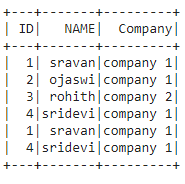Method 1: Using where() function

This function is used to check the condition and give the results

Syntax: dataframe.where(condition)

We are going to filter the rows by using column values through the condition, where the condition is the dataframe condition

Example 1: filter rows in dataframe where ID =1

## Python3

 `# get the data where ID=1``dataframe.where(dataframe.``ID``=``=``'1'``).show()`

Output: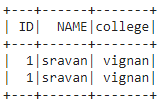Example 2:

## Python3

 `# get the data where name not 'sravan'``dataframe.where(dataframe.NAME !``=` `'sravan'``).show()`

Output: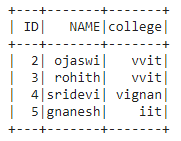Example 3: Where clause multiple column values filtering.

Python program to filter rows where ID greater than 2 and college is vvit

## Python3

 `# filter rows where ID greater than 2``# and college is vvit``dataframe.where((dataframe.``ID``>``'2'``) & (dataframe.college``=``=``'vvit'``)).show()`

Output: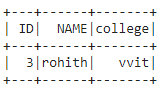Method 2: Using filter() function

This function is used to check the condition and give the results.

Syntax: dataframe.filter(condition)

Example 1: Python code to get column value = vvit college

## Python3

 `# get the data where college is  'vvit'``dataframe.``filter``(dataframe.college``=``=``'vvit'``).show()`

Output: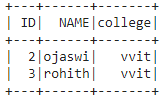Example 2: filter the data where id > 3.

## Python3

 `# get the data where id > 3``dataframe.``filter``(dataframe.``ID``>``'3'``).show()`

Output: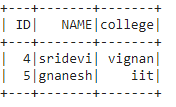Example 3: Multiple column value filtering.

Python program to filter rows where ID greater than 2 and college is vignan

## Python3

 `# filter rows where ID greater``# than 2 and college is vignan``dataframe.``filter``((dataframe.``ID``>``'2'``) &``                 ``(dataframe.college``=``=``'vignan'``)).show()`

Output: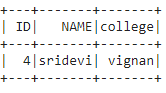Attention geek! Strengthen your foundations with the Python Programming Foundation Course and learn the basics.

To begin with, your interview preparations Enhance your Data Structures concepts with the Python DS Course. And to begin with your Machine Learning Journey, join the Machine Learning – Basic Level Course

My Personal Notes arrow_drop_up